# Cylinder

Go to Surface Area or Volume.

Cylinder Facts

Notice these interesting things: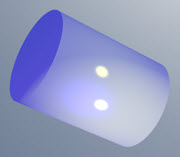• It has a flat base and a
flat top
• The base is the same as the top
• From base to top the shape stays the same
• It has one curved side
• It is not a polyhedron as it has a curved surface
images/poly-gl.js?mode=cylinder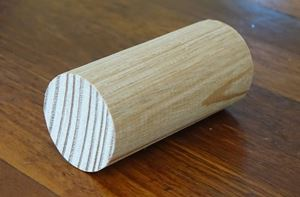A wooden cylinder

An object shaped like a cylinder is said to be cylindrical.

## Oblique Cylinder

When the two ends are directly aligned on each other it is a Right Cylinder otherwise it is an Oblique Cylinder:## Surface Area of a CylinderThe Surface Area has these parts:

• Surface Area of Both Ends = 2 × π × r2
• Surface Area of Side = 2 × π × r × h

Which together make:

Surface Area = 2 × π × r × (r+h)

### Example: h = 7 and r = 2

Surface Area = 2 × π × r × (r+h)
= 2 × π × 2 × (2+7)
= 2 × π × 2 × 9
= 36 π
113.097

Try it yourself: cut some paper so it fits around a cylinder, then unwrap and measure it.

It will be h high and 2πr (the circumference of a circle) long: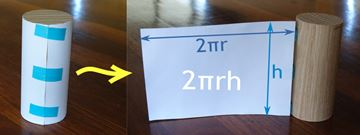Side area = 2πrh

Don't forget the two end bits: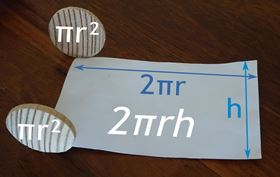Total Surface Area
= 2(πr2) + 2πrh
= 2πr(r+h)

## Volume of a CylinderTo calculate the volume we multiply the area of the base by the height of the cylinder:

• Area of the base: π × r2
• Height: h

And we get:

Volume = π × r2 × h

### Example: h = 7 and r = 2

Volume= π × r2 × h
= π × 22 × 7
= 28 π
87.96

### How to remember: Volume = pizzaImagine you just cooked a pizza.

The radius is "z", and the thickness "a" is the same everywhere ... what is the volume?

Volume =   pi × z × z × a

(we would normally write "pi" as π, and z × z as z2, but you get the idea!)

Play with it here. The formula also works when it "leans over" (oblique) but remember that the height is always at right angles to the base:

And this is why: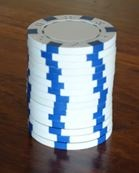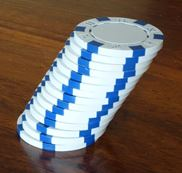The stack leans over, but still has the same volume

## Volume of a Tank

Learn how to find the volume of a partly filled horizontal cylinder like this one:## Volume of a Cone vs CylinderThe volume formulas for cylinders and cones are very similar:

 Volume of a cylinder: π × r2 × h Volume of a cone: 1 3 π × r2 × h

So a cone's volume is exactly one third ( 1 3 ) of a cylinder's volume.

In future, order your ice creams in cylinders, not cones, you get 3 times as much!

## Like a Prism

A cylinder is like a prism with an infinite number of sides, see Prism vs Cylinder.

## It Doesn't Have to Be Circular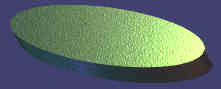Usually when we say Cylinder we mean a Circular Cylinder, but you can also have Elliptical Cylinders, like this one:

And we can have stranger cylinders!

So long as the cross-section is curved and is the same from one end to the other, then it is a cylinder. But the area and volume calculations will be different than shown above.

## More Cylinders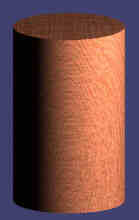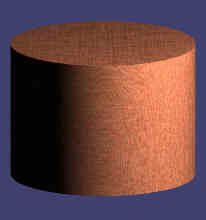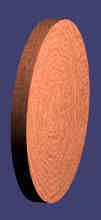"We eat what we can
And what we can't we can"

5495, 5589, 5591, 5592, 869, 870, 3388, 3390, 3387, 3389